### Home > MC1 > Chapter 6 > Lesson 6.1.2 > Problem6-17

6-17.
1. . Simplify each expression. Homework Help ✎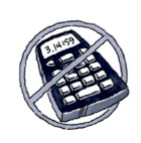1. 6 + (−2)

2. −7 + (−7)

3. 1 − (−4)

4. −6 · 7 + 11 + 1(−3)

5. 5 groups of (−6)

6. −7(−4)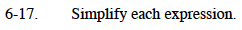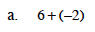4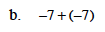−14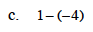Remember that subtracting a negative can be thought of as undoing the adding of a negative.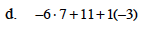Circling terms should make this problem easier.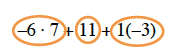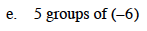This is like saying 5(−6).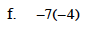28